# Algebra Calculator Tutorial

Step-by-step calculator for algebra problems.

This is a tutorial on how to use the Algebra Calculator, a step-by-step calculator for algebra.

### Solving Equations

First go to the Algebra Calculator main page. In the Calculator's text box, you can enter a math problem that you want to calculate.

For example, try entering the equation 3x+2=14 into the text box.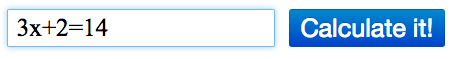After you enter the expression, Algebra Calculator will print a step-by-step explanation of how to solve 3x+2=14.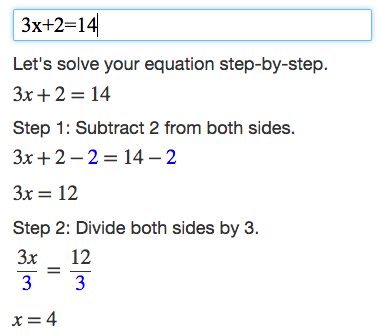### Examples

To see more examples of problems that Algebra Calculator understands, visit the Examples page. Feel free to try them now.

### Math Symbols

If you would like to create your own math expressions, here are some symbols that Algebra Calculator understands:

- (Subtraction)
* (Multiplication)
/ (Division)
^ (Exponent: "raised to the power")

### Graphing

To graph an equation, enter an equation that starts with "y=" or "x=". Here are some examples: y=2x^2+1, y=3x-1, x=5, x=y^2.

To graph a point, enter an ordered pair with the x-coordinate and y-coordinate separated by a comma, e.g., (3,4).

To graph two objects, simply place a semicolon between the two commands, e.g., y=2x^2+1; y=3x-1.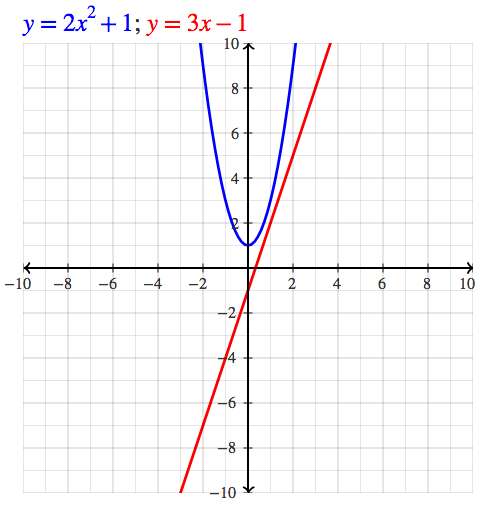### Polynomials

Algebra Calculator can simplify polynomials, but it only supports polynomials containing the variable x.

Here are some examples: x^2 + x + 2 + (2x^2 - 2x), (x+3)^2.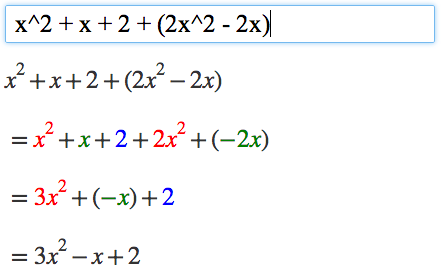### Evaluating Expressions

Algebra Calculator can evaluate expressions that contain the variable x.

To evaluate an expression containing x, enter the expression you want to evaluate, followed by the @ sign and the value you want to plug in for x. For example the command 2x @ 3 evaluates the expression 2x for x=3, which is equal to 2*3 or 6.

Algebra Calculator can also evaluate expressions that contain variables x and y. To evaluate an expression containing x and y, enter the expression you want to evaluate, followed by the @ sign and an ordered pair containing your x-value and y-value. Here is an example evaluating the expression xy at the point (3,4): xy @ (3,4).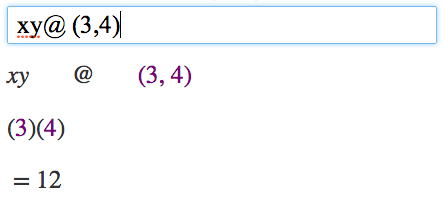### Checking Answers For Solving Equations

Just as Algebra Calculator can be used to evaluate expressions, Algebra Calculator can also be used to check answers for solving equations containing x.

As an example, suppose we solved 2x+3=7 and got x=2. If we want to plug 2 back into the original equation to check our work, we can do so: 2x+3=7 @ 2. Since the answer is right, Algebra Calculator shows a green equals sign.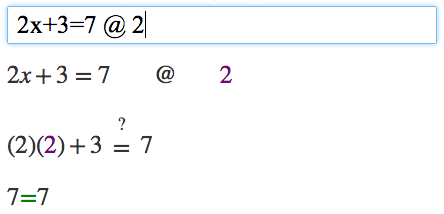If we instead try a value that doesn't work, say x=3 (try 2x+3=7 @ 3), Algebra Calculator shows a red "not equals" sign instead.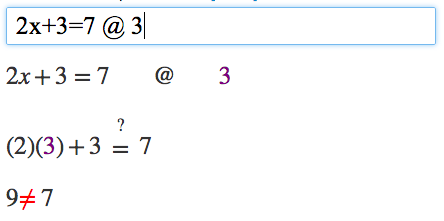To check an answer to a system of equations containing x and y, enter the two equations separated by a semicolon, followed by the @ sign and an ordered pair containing your x-value and y-value. Example: x+y=7; x+2y=11 @ (3,4).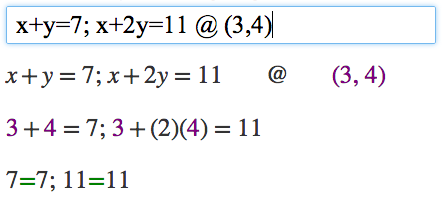### Tablet Mode

If you are using a tablet such as the iPad, enter Tablet Mode to display a touch keypad.